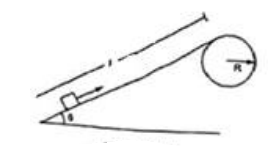# Solve the following :Question:

A particle of mass $m$ is kept on the top of a smooth sphere of radius $\mathrm{R}$. It is given a sharp impulse which imparts it a horizontal speed v. (a) Find the normal force between the sphere and the particle just after the impulse. (b) What should be the minimum value of $v$ for which the particle does not slip on the sphere? (c) Assuming the velocity $v$ to be half the minimum calculated in part, (b) Find the angle made by the radius through the particle with the vertical when it leaves the sphere.Solution:

$\mathrm{N}=\mathrm{mg}-\left(\mathrm{mv}^{2}\right) / \mathrm{R}$

(b) When particle moves with maximum velocity then, $\mathrm{mg}=\left(\mathrm{mv}^{2}\right) / \mathrm{R}$

$\mathrm{mg}=\left(\mathrm{mv}^{2}\right) / \mathrm{R}$

$v=\sqrt{g} R$

(c ) From figure,

$\mathrm{v}_{1}=(\sqrt{g R}) / 2 \ldots \ldots 1$

and

$\left(m v_{2}^{2}\right) / R=m g \cos \theta$

$\mathrm{v}_{2}=(\sqrt{g} \mathrm{R} \cos \theta) \ldots \ldots 2$

Also, change in K.E. = Work done

$\frac{\frac{1}{2}}{m_{2}} v_{2}^{2}-{ }^{\frac{1}{2}} m v_{1}=m g R(1-\cos \theta) \ldots \ldots 3$

from equation 2 and 3

$g R \cos \theta=v_{1}^{2}+2 g R(1-\cos \theta)$

$\theta=\cos ^{-1}\left(\frac{3}{4}\right)$